# Tricky heat calculation from the 1850s

Camomille
Hi everyone !
I'm calling for help, for I have been unable to make sense of 1850s calculus !
In the Mechanical Theory of Heat, R. Clausius introduces the mathematical expression of internal energy by investigating an infinitesimal Carnot cycle (that is to say all volume variations are infinitesimal) and therefore approximates every transformation as linear (cf attached graph).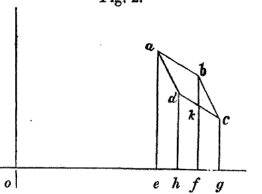Another Carnot cycle (not infinitesimal !), with his notations.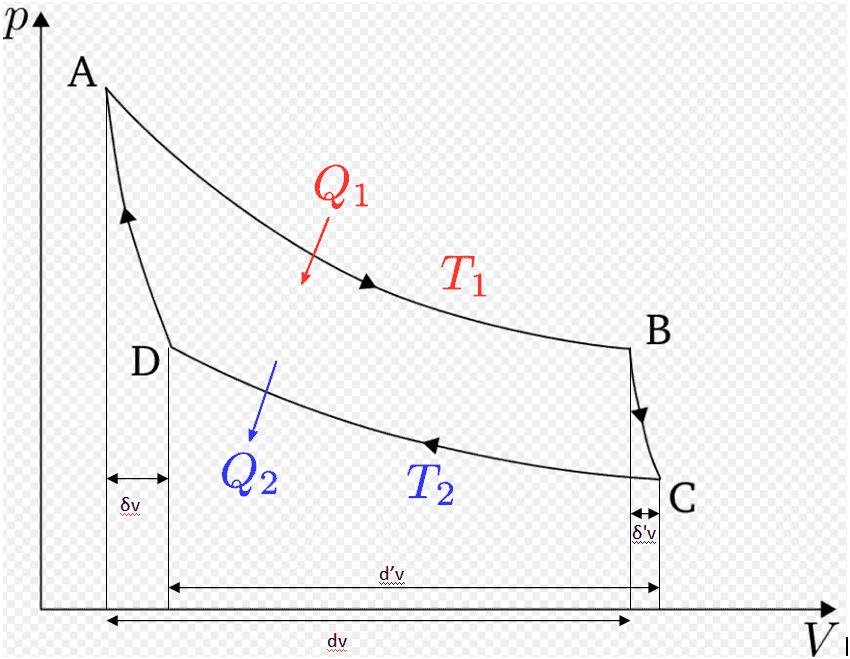He firstly states that Q1=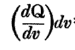, which I can't but agree with. But he got me there :
-Q2 =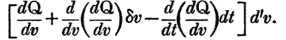(beware of the "-")
Honestly, I have no clue why there should be second derivatives !
The original text for my would-be saviour : Mechanical theory of heat on Google Book

Sorry for the potential mistakes, I'm French AND a math major student !

Mentor
At first glance, I can't figure out what he is doing here. But, if it were me, what I would do would be to do the derivation on my own from scratch (without referring to this). At least I could understand that.

•Camomille
Homework Helper
Gold Member
But he got me there :
-Q2 =View attachment 245845 (beware of the "-")
Honestly, I have no clue why there should be second derivatives !
Clausius’ goal is to prove that heat is not a state variable, contrary to the opinion of some scientists of that time period. In order to follow his argument, it is very important to understand the notation that Clausius is using. I also think that it’s important to understand the “Mathematical Introduction” on pages 1 -13.

The notation ## \frac{dQ}{dv}## associated with a state, ##A##, of the system can be defined operationally as follows. Imagine the system starting in state A. Add a very small amount of heat ##dQ## to the system while keeping the temperature ##t## of the system constant. Let ##dv## be the corresponding change in volume (assumed to be nonzero). The meaning of ## \frac{dQ}{dv}## is simply the ratio of the two quantities ##dQ## and ##dv##. This definition does not require us to think of ##\frac{dQ}{dv}## as a partial derivative of some function ##Q(v, t)##. Clausius’s goal is to show that such a function ##Q(v,t)## does not exist!

The ratio ##\frac{dQ}{dv}## was defined above for the state ##A## since we took state ##A## as the initial state when adding the heat ##dQ##. So, it might have been clearer to write the notation as ##\frac{dQ}{dv}|_A##. Clearly, we can carry out the definition for any initial state, ##S##, of the system to get ##\frac{dQ}{dv}|_S##. Thus, there is a value of ##\frac{dQ}{dv}|_S## at each state ##S##. But, a state is determined by values of ##v## and ##t##. So, you can think of ## \frac{dQ}{dv}## as defining some function ##F(v,t)##. Note that ##F## is a state variable! It has a definite value for each state of the system.

In going isothermally from state ##A## to state##B## in your diagram, the heat added is ##\left ( \frac{dQ}{dv}|_A \right) dv##, where ##dv## is the change in volume when going from ##A## to ##B##. Likewise, if you were to start at ##D## and go isothermally to ##C##, the heat added would be ##\left ( \frac{dQ}{dv}|_D \right) d’v##, where ##d’v## is the change in volume when going from ##D## to ##C##. (The heat removed when going the other way from ##C## to ##D## is just the negative of this.)

The heat added in going from ##D## to ##C## can be expressed as ##F(v_D, t_D) d’v##.

Since ##D## is close to ##A##, we can write ##F(v_D, t_D)## in terms of ##F(v_A, t_A)## to sufficient accuracy as

##F(v_D, t_D) = F(v_A, t_A) + \frac {\partial F}{\partial v}|_A \delta v + \frac {\partial F}{\partial t}|_A (-dt)##. Note that the volume change in going from ##A## to ##D## is ##\delta v## and the temperature change in going from ##A## to ##D## is ##(-dt)## according to Clausius’ definitions of symbols. So, the heat that would be added in going from ##D## to ##C## can be expressed as

## F(v_D, t_D) d’v =\left[ F(v_A, t_A) + \frac {\partial F}{\partial v}|_A \delta v - \frac {\partial F}{\partial t}|_A dt\right] d’v##.

This is essentially the same as Clausius’ expression that you were asking about:Clausius uses the notation ##\frac{dQ}{dv}## for ##\frac{dQ}{dv}|_A = F(v_A, t_A)##.

••nasu and Camomille
Camomille
At first glance, I can't figure out what he is doing here. But, if it were me, what I would do would be to do the derivation on my own from scratch (without referring to this). At least I could understand that.
You're right : I tried but I'm just not used to phycisists' analysis ! I'm used to clear exercices where I just have to find an asymptotic equivalent to prove that a series is converging ! I got lost with all those dv, d'v, dt...Camomille
Clausius’ goal is to prove that heat is not a state variable, contrary to the opinion of some scientists of that time period. In order to follow his argument, it is very important to understand the notation that Clausius is using. I also think that it’s important to understand the “Mathematical Introduction” on pages 1 -13.

The notation ## \frac{dQ}{dv}## associated with a state, ##A##, of the system can be defined operationally as follows. Imagine the system starting in state A. Add a very small amount of heat ##dQ## to the system while keeping the temperature ##t## of the system constant. Let ##dv## be the corresponding change in volume (assumed to be nonzero). The meaning of ## \frac{dQ}{dv}## is simply the ratio of the two quantities ##dQ## and ##dv##. This definition does not require us to think of ##\frac{dQ}{dv}## as a partial derivative of some function ##Q(v, t)##. Clausius’s goal is to show that such a function ##Q(v,t)## does not exist!

The ratio ##\frac{dQ}{dv}## was defined above for the state ##A## since we took state ##A## as the initial state when adding the heat ##dQ##. So, it might have been clearer to write the notation as ##\frac{dQ}{dv}|_A##. Clearly, we can carry out the definition for any initial state, ##S##, of the system to get ##\frac{dQ}{dv}|_S##. Thus, there is a value of ##\frac{dQ}{dv}|_S## at each state ##S##. But, a state is determined by values of ##v## and ##t##. So, you can think of ## \frac{dQ}{dv}## as defining some function ##F(v,t)##. Note that ##F## is a state variable! It has a definite value for each state of the system.

In going isothermally from state ##A## to state##B## in your diagram, the heat added is ##\left ( \frac{dQ}{dv}|_A \right) dv##, where ##dv## is the change in volume when going from ##A## to ##B##. Likewise, if you were to start at ##D## and go isothermally to ##C##, the heat added would be ##\left ( \frac{dQ}{dv}|_D \right) d’v##, where ##d’v## is the change in volume when going from ##D## to ##C##. (The heat removed when going the other way from ##C## to ##D## is just the negative of this.)

The heat added in going from ##D## to ##C## can be expressed as ##F(v_D, t_D) d’v##.

Since ##D## is close to ##A##, we can write ##F(v_D, t_D)## in terms of ##F(v_A, t_A)## to sufficient accuracy as

##F(v_D, t_D) = F(v_A, t_A) + \frac {\partial F}{\partial v}|_A \delta v + \frac {\partial F}{\partial t}|_A (-dt)##. Note that the volume change in going from ##A## to ##D## is ##\delta v## and the temperature change in going from ##A## to ##D## is ##(-dt)## according to Clausius’ definitions of symbols. So, the heat that would be added in going from ##D## to ##C## can be expressed as

## F(v_D, t_D) d’v =\left[ F(v_A, t_A) + \frac {\partial F}{\partial v}|_A \delta v - \frac {\partial F}{\partial t}|_A dt\right] d’v##.

This is essentially the same as Clausius’ expression that you were asking about:

View attachment 245872

Clausius uses the notation ##\frac{dQ}{dv}## for ##\frac{dQ}{dv}|_A = F(v_A, t_A)##.
It's much clearer now ! Thank so much !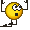I really hadn't understood he was trying to express what you have denoted F(v_d,t_d) in terms of F(v_a,t_a).